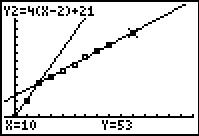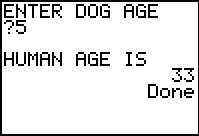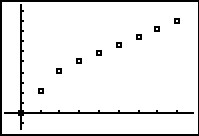•• ##### Device
• TI-83 Plus Family
• TI-84 Plus
•TI-84 Plus CE
• ##### Software

TI Connect™
TI Connect™ CE

# SA and NT: Dog Days or Dog Years?

by Texas Instruments#### Overview

Students will use order pairs, table of values, and a scatter plot to determine a function that represents real world data.

#### Key Steps

•Students will investigate data that takes a dog’s age and converts it into human years. They will analyze the data to determine if the claim that each dog year is equal to 7 human years is supported. Students will first use a dynamic input/output program to determine some ordered pairs of the data.

•Then students will gather information from lists and a scatter plot. They can use the Trace feature to move through the points. Students should see that two linear functions can represent the data.

•After using points to calculate slope and write the equation of a line, students will graph their functions on the scatter plot. They can then use the graph to determine a dog’s age in human years beyond 8 years old.